Published: 15 August 2015

# Experimental validation of a quasi-realtime human respiration detection method via UWB radar

Shiyou Wu1
Zhenghuan Xia2
Kai Tan3
Jie Chen4
Shengwei Meng5
Guangyou Fang6
Hejun Yin7
1, 3, 4, 6Key Laboratory of Electromagnetic Radiation and Sensing Technology Institute of Electronics, Chinese Academy of Sciences, Beijing, China
2State Key Laboratory of Space-Ground Integrated Information Technology, Beijing, China
2Space Star Technology Co., Ltd., Beijing, China
3University of Chinese Academy of Sciences, Beijing, China
5Harbin Institute of Technology, Beijing, China
7Chinese Academy of Sciences, Beijing, China
Corresponding Author:
Shiyou Wu
Views 80

#### Abstract

In this paper, we propose a quasi-realtime human respiration detection method via UWB radar system in through-wall or similar condition. With respect to the previous proposed automatic detection method, the new proposed method assures competitive performance in the human respiration motion detection and effective noise/clutter rejection, which have been proved by experimental results in actual scenario. This new method has also been implemented in a UWB through-wall life-detection radar prototype, and its time consuming is about 2 s, which can satisfy the practical requirement of quasi-realtime for through-wall sequential vital sign detection. Therefore, it can be an alternative for through-obstacles static human detection in antiterrorism or rescue scenarios.

## 1. Introduction

In this paper, we apply a UWB pulse radar system in through-obstacles human respiration detection and present the experimental validation of the new proposed quasi-realtime detection method that aims at improving the performance of respiration detection. Compared to the previous proposed method , non-stationary clutter will be suppressed effectively in the new method. Meanwhile, the time consuming is about 2 s so that the quasi-realtime through-wall human respiration detection is possible. This feature might also be suitable for through-obstacles static human detection in antiterrorism.

The rest of this paper is organized as follows. In section 2, the overall radar system is described both in measurement device and detection procedure aspects, while the experimental results are reported in section 3. Finally, section 4 concludes this paper.

## 2. UWB through-wall life-detection radar system

### 2.1. UWB time domain respiratory signal model

Referring to [11, 12], for impulse UWB radar system, the received signal $S\left(t\right)$ with the respiration motion of one human subject can be expressed as:

1
$S\left(t\right)=\sum _{n=0}^{N-1}u\left(t-nT-{\tau }_{r}\right)\mathrm{*}{h}_{r}\left(t\right)+\sum _{n=0}^{N-1}\sum _{p=1,p\ne r}^{P}u\left(t-nT-{\tau }_{p}\right)\mathrm{*}{h}_{p}\left(t\right)+\gamma \left(t\right)+\omega \left(t\right),$

where “$\mathrm{*}$” represents convolution. $u\left(t\right)$ is the transmitted signal, $t$ represents fast-time, slow-time is discrete with $nT$, on which the reflected signal is received, $N$ is slow-time sampling points, $n=\text{0,}\text{}\text{1,}\text{…}\text{,}\text{}N-1$ and $T$ is the effective pulse repetition time (PRT). ${h}_{r}\left(t\right)$ is the respiratory response of the human subject, and ${h}_{p}\left(t\right)$ is the joint impulse response of transmitting antenna, receiving antenna, and $P-1$ static objects. ${\tau }_{r}$ and ${\tau }_{p}$ are the propagation time-delay of the human subject and the $p$th object, respectively. $\gamma \left(t\right)$ is the non-stationary inference, and $\omega \left(t\right)$ is the additive white Gaussian noise.

In order to avoid frequency aliasing and range ambiguities, $T$ should be set to satisfy Nyquist sampling theorem and guarantee that all the reflected signals of objects are received in one PRT . The discrete signal of all the reflected signals can be expressed as 2-D (fast-time and slow-time) $M$×$N$ matrix $S\left(m,n\right)$:

2
$S\left(m,n\right)=h\left(m,n\right)+c\left(m,n\right)+\gamma \left(m,n\right)+\omega \left(m,n\right),$

where $M$ is fast-time sampling points, $m=\text{0,}\text{}\text{1,}\text{…}\text{,}\text{}M-1$, and $N$ is slow-time sampling points, $n=\text{0,}\text{}\text{1,}\text{…}\text{,}\text{}N-1$. Every term in Eq. (2) corresponds to that in Eq. (1).

### 2.2. Quasi-realtime detection procedure

Here, we propose an improved version of the previous method , referred to as the quasi-realtime human respiration detection (QHRD) method in the following. The proposed QHRD method consists of three main steps: ${A}^{\text{'}}$) Pre-Processing, ${B}^{\text{'}}$) FFT in Slow-time Convolution Dimension and ${C}^{\text{'}}$) Spectrum Weighted Accumulation (SWA) method, as illustrated in Fig. 1(a). In the previous method, there are also three main steps: $A$) Pre-Processing, $B$) FFT in Slow-time Dimension and $C$) Constant False Alarm Rate Clustering (CFAR-Clustering) method, as shown in Fig. 1(b). Obviously, the step ${A}^{\text{'}}$ is developed from the step $A$. The adaptive background subtraction (ABS) method in  is used to remove the static clutter. The linear trend suppression (LTS) method in  is adopted to suppress the non-static clutter and linear trend in slow-time dimension caused by the data acquisition accompanying with the instability of radar time-base. The advance normalization (AN) method in  is used for the enhancement of the weak human respiratory signal. A moving average filter is also applied both along the fast-time and slow-time dimensions to eliminate and suppress the high-frequency clutter interferences .

As shown in Fig. 1(a), the 2-D $M$×$N$ matrix $S\left(m,n\right)$ in Eq. (2) is taken as the input of the step ${A}^{\text{'}}$, the corresponding output matrix is named as $\stackrel{~}{S}\left(m,n\right)$. The aim of all the above methods performed in the step ${A}^{\text{'}}$ is to further improve the signal to noise/clutter ratio (SNCR) of $\stackrel{~}{S}\left(m,n\right)$. In the following, the step ${B}^{\text{'}}$ and step ${C}^{\text{'}}$ in the QHRD method different from the previous method will be given out.

Fig. 1a) The flowchart of the QHRD method, b) the flowchart of the previous method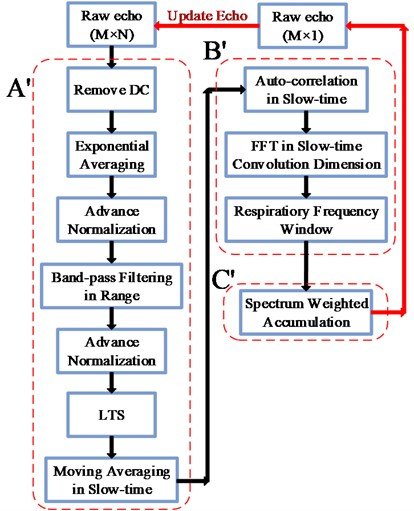a)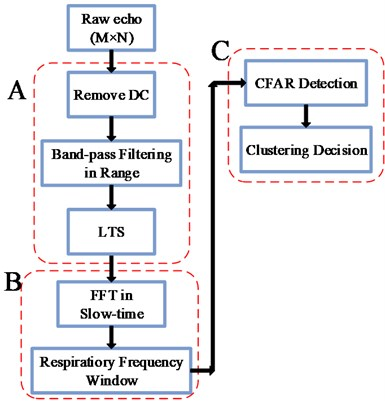b)

### 2.2.1. FFT in slow-time convolution dimension

Generally, there is a strong correlation among the values of the deterministic signal in different time instant. Otherwise, the correlation of the interference noise is always weak because of the high randomness. Based on this characteristic, the deterministic signal can be extracted from the interference noise. For the $\mathrm{m}\mathrm{t}\mathrm{h}$ slow-time signal ${x}_{m}\left(\tau \right)$ in $\stackrel{~}{S}\left(m,n\right)$ added with zero mean random noise, the contribution from the noise can be very small in the auto-correlation function ${R}_{{x}_{m}}\left(\tau \right)$, which mainly consists of the characteristics of the deterministic signal like DC, the amplitude and frequency of the periodic components and so on. For the non-periodic noise, the auto-correlation function is prone to zero:

3
${R}_{{x}_{m}}\left(\tau \right)=E\left[{x}_{m}\left({\tau }_{1}\right){x}_{m}\left({\tau }_{2}\right)\right],$

where ${0\le \tau }_{1}\text{,}$${\tau }_{2}\le N-1$ and $\tau ={\tau }_{1}-{\tau }_{2}\text{.}$ The periodic component and its harmonic components of the slow-time signal ${x}_{m}\left(\tau \right)$ are still preserved and expressed as ${R}_{{x}_{m}}\left(\tau \right)$. ${R}_{{x}_{m}}\left(\tau \right)$ can be discretized and taken as the $m$th row vector of the 2-D (fast-time and slow-time convolution) $M$×$\left(2N-1\right)$ matrix ${R}_{x}\left(m,n\right)$, where $M$ is fast-time sampling points and $m=\text{0,}\text{}\text{1,}\text{…}\text{,}\text{}M-1$, $2N-1$ is convolution length and $n=\text{0,}\text{}\text{1,}\text{…}\text{,}\text{}2N-2$. To extract the respiratory frequency, the FFT is performed for ${R}_{x}\left(m,n\right)$ in slow-time convolution dimension, and its resultant matrix is the output range-frequency matrix ${\stackrel{~}{R}}_{x}\left(m,n\right)$ of the step ${B}^{\text{'}}$ shown in Fig. 1(a). According to the fact that the respiratory frequency is confined in a narrow frequency range, one selected frequency window ranging from 0.05 to 1 Hz is added to eliminate high frequencies, high harmonics, and very low frequencies in ${\stackrel{~}{R}}_{x}\left(m,n\right)$.

### 2.2.2. Spectrum weighted accumulation (SWA) method

According to the implementation steps mentioned in Fig. 1(b), the CFAR-Clustering method is performed to extract respiratory frequency. The CFAR method based on the characteristic of vital sign in the FFT resultant matrix is used to detect vital sign in low SNCR conditions automatically. The decision whether the vital sign exists or not and the estimation of the detailed vital information are implemented in the clustering decision method. However, the CFAR moving energy window calculation in the CFAR method and the vital sign points of interest (VSPOI) identification in the clustering decision method are always time consuming. Furthermore, the detection probability of the vital sign is highly depended on the selected local signal to background energy ratio ${T}_{r}$, which is empirically determined to be greater than one requiring that the energy of the vital sign is stronger than that of background.

Here, a new spectrum weighted accumulation (SWA) method is proposed for the vital sign extraction without any thresholds, the empirical adjustment of which might decrease the practicability of any detection method in actual scenarios. Define the non-weighted spectrum accumulation signal $\stackrel{~}{v}\left(n\right)$, which can be expressed as:

4
$\stackrel{~}{v}\left(n\right)=\sum _{m={m}_{1}}^{{m}_{2}}{\left|{\stackrel{~}{r}}_{m}\left(n\right)\right|}^{2},$

where $n=\text{0, 1,}\text{…}\text{,}\text{}2N-2$ and ${\stackrel{~}{r}}_{m}\left(n\right)$ is the $m$th FFT result in range-frequency matrix ${\stackrel{~}{R}}_{x}\left(m,n\right)$, which can be used to extract the periodic component. Although the spectral amplitude of the periodic component of respiration motion is always small in ${\stackrel{~}{r}}_{m}\left(n\right)$, it can be strengthened in the spectrum accumulation signal $\stackrel{~}{v}\left(n\right)$ to improve the SNCR. Whether the vital sign exists in the range ${\left[m}_{1}\text{,}\text{}{m}_{2}\right]$ or not is hard to decide, so the values of ${m}_{1}$ and ${m}_{2}$ are generally selected as $0$ and $M-1$ respectively. As the spectral amplitude of near-zero frequency component can also be strengthened in $\stackrel{~}{v}\left(n\right)$, the weighted spectrum accumulation signal ${\stackrel{~}{v}}_{w}\left(n\right)$ is present to further remove the interference from the near-zero frequency components:

5
${\stackrel{~}{v}}_{w}\left(n\right)=\sum _{m={m}_{1}}^{{m}_{2}}w\left(n\right)\bullet {\left|{\stackrel{~}{r}}_{m}\left(n\right)\right|}^{2}.$

The weighted factor $w\left(n\right)$ is defined as:

6
$w\left(n\right)=\frac{n}{2N-1}\bullet {F}_{s}-\frac{{F}_{s}}{2},$

where $n=\text{0, 1,}\text{…}\text{,}\text{}2N-2$, ${F}_{s}=N/{T}_{M}$ is the slow-time scanning rate and ${T}_{M}$ is the total measured time, $N$ is slow-time sampling points. Here, the weighted factor $w\left(n\right)$ is selected as the respiratory frequency window. Based on the weighted spectrum accumulation signal ${\stackrel{~}{v}}_{w}\left(n\right)$, it is easy to extract the respiratory frequency by finding the peak value.

Assuming the respiratory frequency of a human being is invariant during the short measured time ${T}_{M}$, there is a one-to-one relationship between the maximums of the ${\stackrel{~}{v}}_{w}\left(n\right)$ and the human being in a limited respiratory frequency window, which is set from 0.05 to 1 Hz according to the prior range of respiratory frequency. The index ${i}_{r}$ corresponding to the peak value of the ${\stackrel{~}{v}}_{w}\left(n\right)$ equals to the index in the weighted factor $w\left(n\right)$, so the respiratory frequency ${f}_{r}$ of the human being is:

7
${f}_{r}=w\left({i}_{r}\right),$
8
${i}_{r}=\mathrm{arg}\underset{n}{\mathrm{max}}\left\{{\stackrel{~}{v}}_{w}\left(n\right)\right\},\mathrm{}\mathrm{}\mathrm{}\mathrm{}\mathrm{}N\le n\le 2N-1.$

When the possible respiratory frequency ${f}_{r}$ is extracted from the ${\stackrel{~}{v}}_{w}\left(n\right)$ and $w\left(n\right)$, the corresponding range profile $y\left(m\right)$ of the vital sign in ${\stackrel{~}{R}}_{x}\left(m,n\right)$ can be obtained:

9
$y\left(m\right)={\stackrel{~}{R}}_{x}\left(m,{i}_{r}\right),$

where $M$ is fast-time sampling points and $m=\text{0, 1,}\text{…}\text{,}M-1$. Similar to the respiratory frequency ${f}_{r}$, the index ${j}_{r}$ corresponding to the peak value of the $y\left(m\right)$ indicates the range location ${L}_{r}$ of the vital sign:

10
${j}_{r}=\mathrm{}\mathrm{arg}\underset{m}{\mathrm{max}}\left\{y\left(m\right)\right\},$
11
${L}_{r}={j}_{r}\bullet \frac{{L}_{\mathrm{m}\mathrm{a}\mathrm{x}}-{L}_{\mathrm{m}\mathrm{i}\mathrm{n}}}{M-1},$

where ${L}_{\mathrm{m}\mathrm{a}\mathrm{x}}$ and ${L}_{\mathrm{m}\mathrm{i}\mathrm{n}}$ are the maximum and minimum distance to be detected respectively. Generally, ${L}_{\mathrm{m}\mathrm{i}\mathrm{n}}$ and ${L}_{\mathrm{m}\mathrm{a}\mathrm{x}}$ can be set to $0$ and ${\left(M-1\right)c\delta }_{t}/2$. Here, ${\delta }_{t}$ is the fast-time sampling interval and $c$ is the propagation speed of the electromagnetic wave.

### 2.3. Update echo for sequential detection

The proposed QHRD method implemented in Matlab takes about 2s to obtain the results of the vital sign. Therefore, it can improve the performance of quasi-realtime through-wall radars employed in emergency or law enforcement operations. According to the flowchart in Fig. 1(a), the 2D $M$×$N$ raw echo matrix $S\left(m,n\right)$ in the detection procedure is updated by a new 1D $M$×$1$ raw echo $s\left(m\right)$. This procedure has been adopted in our Through-wall Life-Detection Radar prototype (see Fig. 2), and offered the sequential range location and respiratory frequency of a human being.

### 2.4. Measurement device

As shown in Fig. 2, the radar prototype employed in human respiration detection was designed by the research group of Key Laboratory of Electromagnetic Radiation and Sensing Technology, Institute of Electronics, Chinese Academy of Sciences. This radar prototype packages two UWB antennas in a 45 cm×22 cm×45 cm box and is operated by a wireless personal digital assistant. One antenna is used for the transmitter, and the other is for the receiver. The key parameters of the radar prototype are shown in Table 1.

Table 1Parameters of the radar prototype

 Parameters Value Operating mode Impulse Centre frequency 400 MHz Amplitude of transmitted signal 50 V Pulse repeated frequency (PRF) 600 KHz Average number (${N}_{A}$) 128 Sampling time window 81 ns Sampling points ($M$) 2048 or 4096 Antenna gain 5-7 dBi Input bandwidth of ADC 2.3 GHz ADC sampling rate 500 MHz Data bits 12 bits Dynamic range of receiver 72 dB

## 3. Experiment and result analysis

The measurement setups for human respiration detection in through-wall or similar condition are shown in Fig. 2. Two experiments were conducted in the through-wall condition, and the third experiment was carried out in artificial-ruins condition. The latter two experiments were designed for the sequential detection. In the first experiment, the echoes were acquired with a male volunteer performing as the test human subject standing at several meters behind a concrete wall, which is shown in Fig. 2(a). The test human subject was straightly facing to the wall. The thickness and the measured average dielectric constant of the concrete wall were 24 cm and 4.93, respectively. In the second experiment, as shown in Fig. 2(b), the test human subject seated statically 2.5 m from the wall. To simulate the actual rescue scenario after earthquake, in the third experiment as shown in Fig. 2(c), the test human subject was lying about 30cm under a broken concrete building structure with the thickness of about 1m.

Fig. 2The measurement setup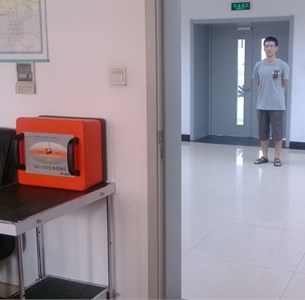a) The test human subject stood behind a concrete wall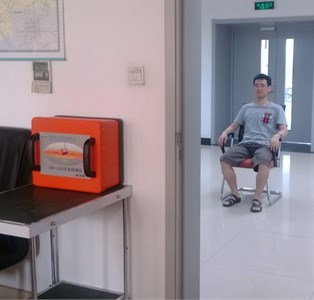b) The test human subject seated statically behind the wall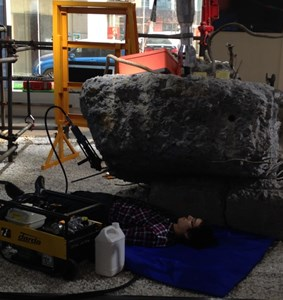c) The test human subject lied under a broken concrete building structure

### 3.1. Performance of the detection procedure

To compare the performances of the two methods shown in Fig. 1, the corresponding results are illustrated and analyzed in the following.

The quasi-periodic slow-time signals representing the respiration of the test human subject are depicted by the red dotted frame in the resultant matrix processed by the step $A$ (see Fig. 3(a)). However, the quasi-periodic respiratory signals cannot be seen clearly. This drawback directly decreases the detection probability of the subsequent step $B$ and step $C$ in the Fig. 1(b). Similarly, the resultant matrix after performing the step ${A}^{\text{'}}$ in Fig. 1(a) is shown in Fig. 3(b).

Fig. 3a) Resultant matrix corresponding to the step A, b) resultant matrix corresponding to the step A', c) range-frequency matrix obtained from the step B, d) range-frequency matrix obtained from the step B'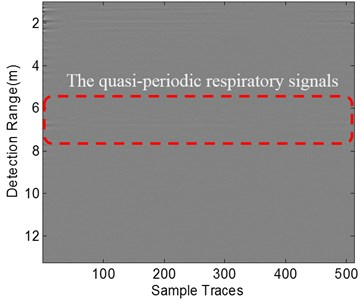a)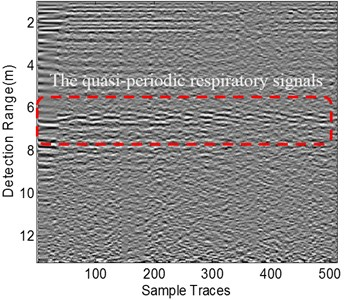b)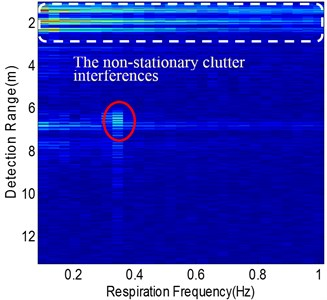c)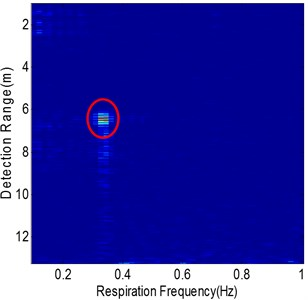d)

Fig. 4Resultant matrices in the specific scenario where a moving person appeared in the front of the test human subject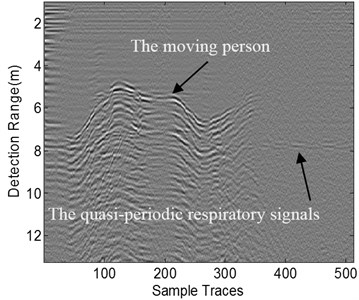a) Resultant matrix corresponding to the step $A$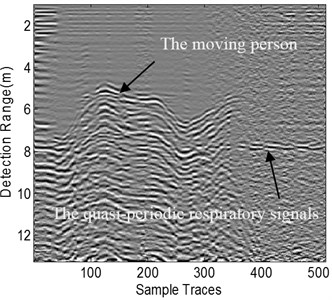b) Resultant matrix corresponding to the step ${A}^{\mathrm{\text{'}}}$

It is obviously noted that the quasi-periodic respiratory signals from the test human subject (depicted by the red dotted frame) can be distinguished easily. Subsequently, as the output of step $B$ and step ${B}^{\text{'}}$ in Fig. 1, the range-frequency matrices are present in Fig. 3(c) and Fig. 3(d) respectively. Although the vital sign of the test human subject in the range-frequency matrix (marked by a red ellipse in Fig. 3(c)) is visible, the range location and respiratory frequency of the vital sign can be hardly extracted due to the low SNCR condition caused by the non-stationary clutter interferences (marked by white dotted frame in Fig. 3(c)). Considering no other moving targets appear in the detection scenario, the non-stationary clutter is mainly brought from the instability of the radar system, but this might not be the only reason. As shown in Fig. 3(d), the non-stationary clutter interferences have been removed dramatically in the resultant matrix obtained from the step ${B}^{\text{'}}$ in Fig. 1(a). Obviously, the background around the vital sign is cleaner than that in Fig. 3(c), which makes the extraction of the vital sign easier.

Fig. 5a) Range-frequency matrix obtained from the step B, b) range-frequency matrix obtained from the step B', c) Respiratory spectrum signals of range-frequency matrix b) and its normalized spectrum weighted accumulation signal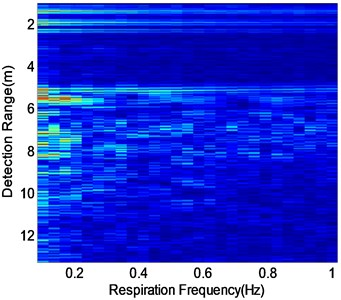a)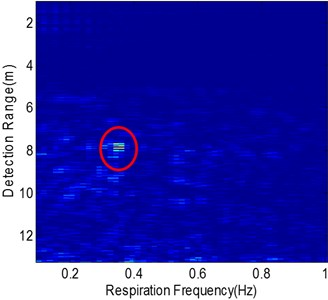b)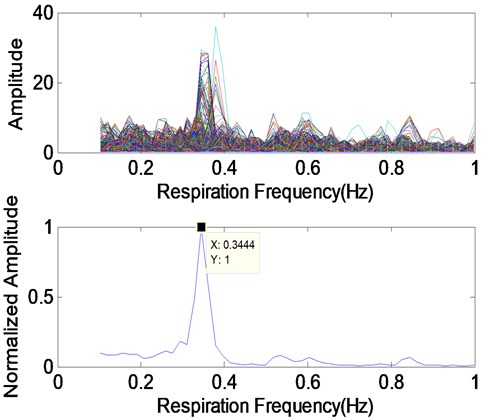c)

Considering the specific scenario where one moving person appeared in the front of the test human subject, the non-stationary clutter interference is strong as shown in Fig. 4(a) and the quasi-periodic respiratory signals are covered when the moving person is nearby. After performing the step ${A}^{\text{'}}$ in Fig. 1(a), the quasi-periodic respiratory signals are strengthened in Fig. 4(b). However, the non-stationary clutter interference caused by the moving person cannot be eliminated. The two range-frequency matrices obtained from the step $B$ and step ${B}^{\text{'}}$ are shown in Fig. 5(a) and Fig. 5(b), respectively. It is noted that the step ${A}^{\text{'}}$ and the step ${B}^{\text{'}}$ of the QHRD method play an important role in eliminating the strong interference from the moving person. Compared to Fig. 5(a), the cleaner background in Fig. 5(b) is remarkable, and the vital sign of the test human subject marked by the red ellipse can be found obviously. In Fig. 5(c), the top plot shows the FFT results of the slow-time signals in Fig. 5(b) for all fast-time sampling points and the respiratory frequency of the test human subject is uncertain. The bottom plot of Fig. 5(c) gives out the normalized weighted spectrum accumulation signal defined as Eq. (5), and the desired respiratory frequency can be obtained clearly and its value is 0.34 Hz.

As mentioned above, the proposed QHRD method improves the previous method in reducing the non-stationary clutter interference, cleaning the background and distinguishing the vital sign in range-frequency matrix effectively.

### 3.2. Sequential detection

According to Section II-C, the sequential results consisting of the detection range location and respiratory frequency of the test human subject are shown in Fig. 6 and Fig. 7, corresponding to measurement setups in Fig. 2(b) and Fig. 2(c) respectively. In Fig. 2(b), the acquired total trace number of the raw echoes is 1700 during the measured time of about 97 seconds. Similarly, the total trace number is 930 and the measured time is about 53 seconds in Fig. 2(c). Before the first implementation of the proposed QHRD method, the measured time needed for the collection of the raw echo matrix $S\left(m,n\right)$ is about 29 s, which is determined by the scanning rate of the radar prototype. Therefore, the first time-consuming of the proposed QHRD method is about 31 seconds, then it might keep a constant value (about 2 seconds).

In Fig. 6, during the measured time ranging from about 29 seconds to 97 seconds, the status (range location and respiratory frequency) of the test human subject is detected in quasi-realtime, the mean values of which are 2.58 m and 0.32 Hz respectively. Thus, it can be seen that the proposed QHRD method can give out the accordant status of the test human subject sequentially and make the quasi-realtime monitoring of a static human being possible in through-wall condition.

Fig. 6The sequential results consisting of the range location a) and respiratory frequency b) of the test human subject in Fig. 2(b) during the measured time ranging from about 29 s to 97 s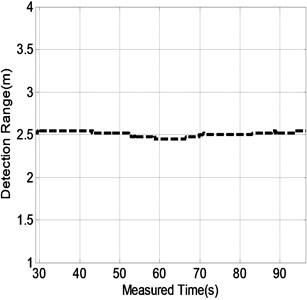a)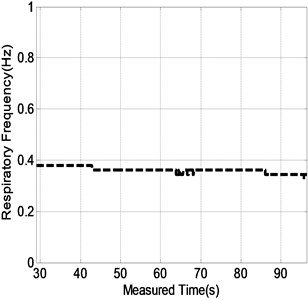b)

Further, the promising results of the proposed QHRD method in the artificial earthquake rescue scenario (see Fig. 2(c)) are shown in Fig. 7. The range-frequency matrix obtained from the step ${B}^{\text{'}}$ at the measured time of 29 s is shown in Fig. 7(a). It is easy to extract the vital sign of the test human subject based on the clean background. The sequential status of the vital sign of the test human subject is updated every 2 seconds from the measured time of 29 seconds to 53 seconds, which are shown in Fig. 7(b) and Fig. 7(c). Likewise, the mean values of the detected status are 1.37 m and 0.44 Hz respectively, which are accordant with the practical status of the test human subject. Therefore, the application of the proposed QHRD method in earthquake rescue is feasible.

Fig. 7a) The Range-frequency matrix obtained from the step B' at the measured time of 29 s. The sequential results consisting of the range location b) and respiratory frequency b) of the test human subject in Fig. 2(c) during the measured time ranging from about 29 s to 53 s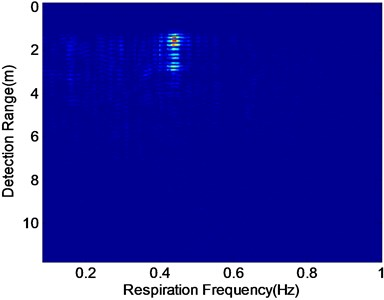a)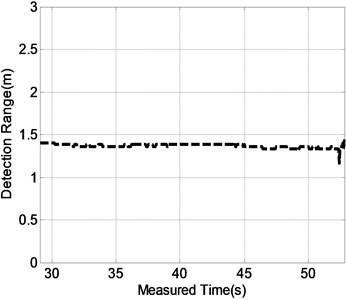b)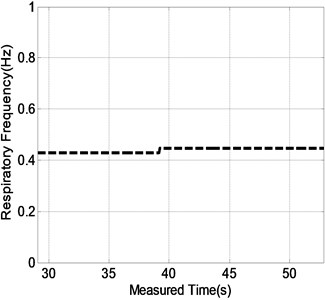c)

## 4. Conclusion

We have proposed a QHRD method for sequential human respiration detection in through-wall or similar condition and provided experimental validations of its capability. The results show that the step ${A}^{\text{'}}$ and ${B}^{\text{'}}$ provide excellent suppression of static/non-static clutter and higher SNCR both in the weighted spectrum accumulation signal and range-frequency matrix. The “cleaner” range-frequency matrix makes the extraction of the vital sign easier. The time-consuming is about 2 s so that the quasi-realtime sequential human respiration detection is possible and suitable for through-obstacles static human detection in antiterrorism or rescue scenarios.

08 June 2015
Accepted
02 August 2015
Published
15 August 2015
SUBJECTS
Oscillations in biomedical engineering
Keywords
quasi-realtime
human respiration detection